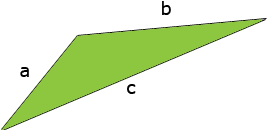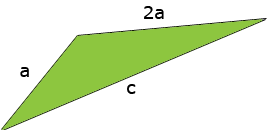A triangle is such that its medium side is twice as long as its shortest side, and its longest side is three times as long as its shortest side. The perimeter of the triangle is 54 yards. What are the lengths of the three sides? Hi, I gave names to the lengths of the sides of the triangle a is the length of the short side in yards, b is the length of the medium side in yards, and c is the length of the long side in yards.The medium side is twice as long as the shortest side so the medium side has length 2a. Thus in my diagram I don't need the letter b since b = 2a.Can you see now how to eliminate the letter c in the diagram by writing c in terms of a? Once you do then you know that the sum of the lengths of the three sides is 54 yards. Penny